# Context help

### The tiling

The tiling is a regular filling of the plane, done with a single figure.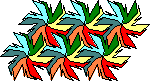The basic figure is the tile: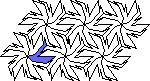The fundamental set of tiles is a group of several tiles which allows to do the tiling in 2 directions of translations: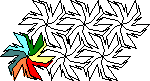### Fundamental set of tiles

The fundamental set of tiles is a group of several tiles which allows to do the tiling in 2 directions of translations.### Tile

The tile is the basic element for a tilingAs a tile is in contact with another, each part of its contour appears twice.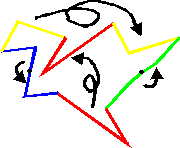### Group of displacement

Each type of tiling is associated to a group of displacement of the plane. This group is made of the displacement allowing to go from one tile to another.

The displacements are:

The groups associated to a different type of tiling are always the results of a finite number of displacements, within which are 2 translations.

### Translations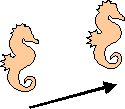In a translation, you displace the figure without turning it over or upside down.
The translation is represented by an arrow called vector.
The 2 translations associated to each tiling allow to go from one fundamental set of tiles to another.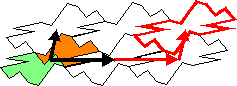### Rotations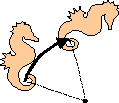In a rotation, the figure turns around a point called center.
It is also necessary to know the angle of rotation.
The rotations associated to a tiling always have their angles being equal to:

• 1/6 of rotation and multiple angles (2/6=1/3, 3/6=1/2, 4/6=2/3 and 5/6)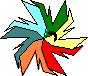• 1/4 of rotation and multiple angles (2/4=1/2 and 3/4)• 1/3 of rotation and multiple angles (2/3)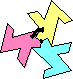• 1/2 of rotation (these are central symmetries)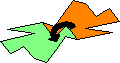The centers of rotation are always on the contour of the tile: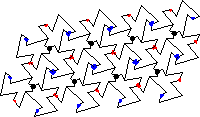### Central symmetries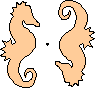A central symmetry is a rotation the angle of which is a 1/2 turn.

### Axial symmetries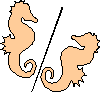In an axial symmetry, the figure is turned around an axis.
When a tiling has an axis of symmetry, a part of the contour of the tile is along this axis and cannot be distorted.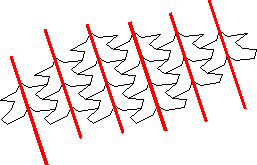The tilings with several directions of angles of symmetry, often have a fixed contour: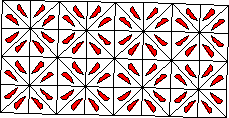### Glide reflections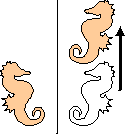You can get a glide reflection if you compose an axial symmetry with a translation the vector of which is parallel to the axis of symmetry.

### Group

A group is a set (let us call it G) with a law of internal composition (if x and y are parts of G , x¤y too) associative (x ¤ y) ¤ z = x ¤ (y ¤ z),We can then write x¤y¤z) as a neutral element exists (e is the neutral element if, for each x, we’ve got x ¤ e = e ¤ x = x) and if each element has an inverse (reciprocal) (for each x , y exists as x ¤ y = y ¤ x = e).

Examples: * the integers (positive or negative) form a group for addition (the neutral element is zero and the opposite (additive inverse) of 5 is -5 * however, positive integers , don’t form a group for addition * the displacements of the plane form a group for composition.

### Composition

The composition of two displacements of the plane consists in doing two displacements one after the other.

Example:

• The composition of two translations is a translation: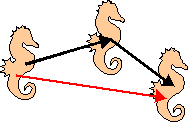• The composition of a translation and a rotation is a rotation: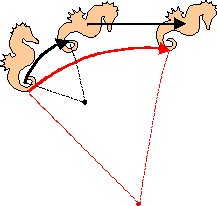• The composition of two axial symmetries is a translation or a rotation: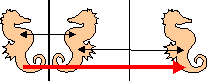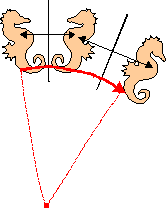# The 17 types of tilings

### (asymmetric parallelogramic)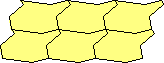The fundamental set of tiles is made of a single tile: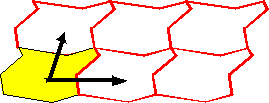The group of displacement is generated by only two translations.

The contour of the tile has got 2 parts: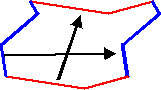### (symmetric parallelogramic)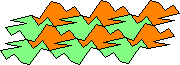The fundamental set of tiles is made of 2 tiles: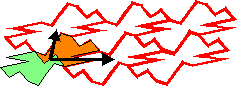The group of displacement is generated by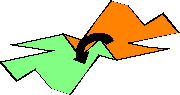The contour of the tile has got 4 parts: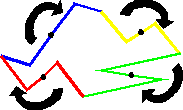### (hexagonal 3-rotative)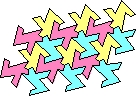The fundamental set of tiles is made of 3 tiles and both the vectors form an equilateral triangle: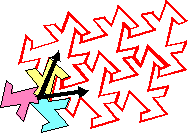The group of displacement is generated by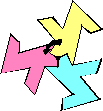The contour of the tile has got 3 parts: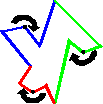### (square 4-rotative)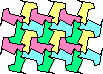The fundamental set of tiles is made of 4 tiles, and both the vectors form a half square: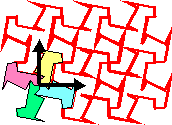The group of displacement is generated by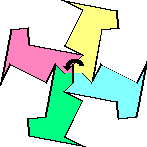The contour of the tile has got 3 parts: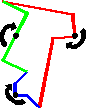### (hexagonal 6-rotative)The fundamental set of tiles is made of 6 tiles, and both the vectors form an equilateral triangle:The group of displacement is generated by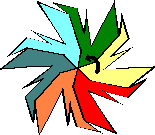The contour of the tile has got 3 parts: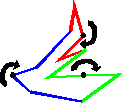### (gliding rectangular)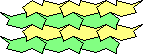The fundamental set of tiles is made of 2 tiles, and both the vectors form a half rectangle: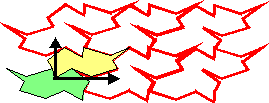The group of displacement is generated by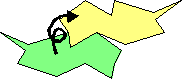The contour of the tile has got 3 parts: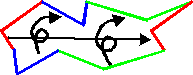### (bi-gliding rectangular)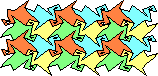The fundamental set of tiles is made of 4 tiles, and both the vectors form a half rectangle: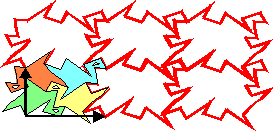The group of displacement is generated by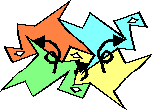The contour of the tile has got 4 parts:### (mono-symmetric rectangular)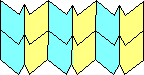The fundamental set of tiles is made of 2 tiles, and both the vectors form a half rectangle: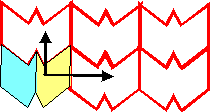The group of displacement is generated by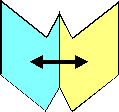The contour of the tile has got 2 parts: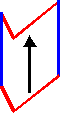### (mono-symmetric rhombic)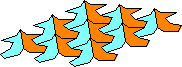The fundamental set of tiles is made of 2 tiles, and both the vectors form an isosceles triangle: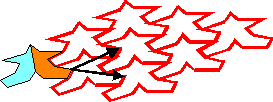The group of displacement is generated by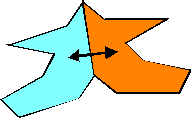The contour of the tile has got 3 parts: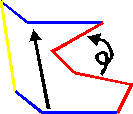### (symmetric gliding

rectangular)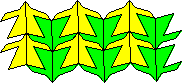The fundamental set of tiles is made of 4 tiles, and both the vectors form a half rectangle: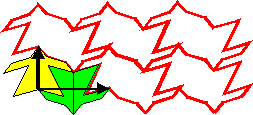The group of displacement is generated by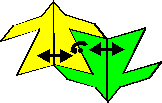The contour of the tile has got 3 parts: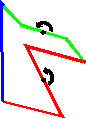### (bi-symmetric rectangular)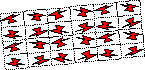The fundamental set of tiles is made of 4 tiles, and both the vectors form a half rectangle: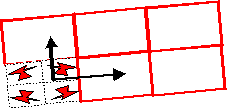The group of displacement is generated by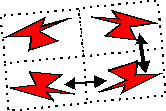The contour of the tile is a rectangle.

### (bi-symmetric rhombic)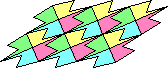The fundamental set of tiles is made of 4 tiles, and both the vectors form an isosceles triangle: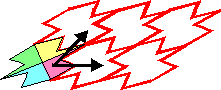The group of displacement is generated by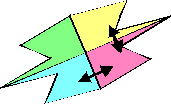The contour of the tile has got 3 parts: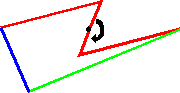### (gliding 4-rotative square)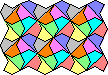The fundamental set of tiles is made of 8 tiles, and both the vectors form a half square:The group of displacement is generated by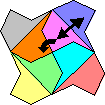The contour of the tile has got 2 parts: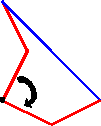### (tri-symmetric hexagonal)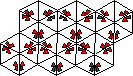The fundamental set of tiles is made of 6 tiles, and both the vectors form an equilateral triangle: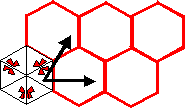The group of displacement is generated byThe contour of the tile is an equilateral triangle.

### (symmetric 3-rotative hexagonal)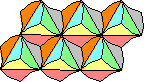The fundamental set of tiles is made of 6 tiles, and both the vectors form an equilateral triangle: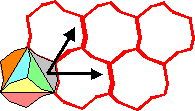The group of displacement is generated by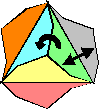The contour of the tile has got 2 parts: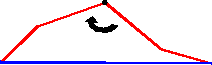### (totally symmetric square)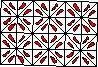The fundamental set of tiles is made of 8 tiles, and both the vectors form a half square: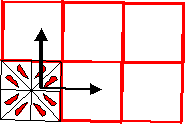The group of displacement is generated by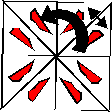The contour of the tile is a right and isosceles triangle (half square).

### (totally symmetric hexagonal)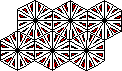The fundamental set of tiles is made of 12 tiles, and both the vectors form an equilateral triangle: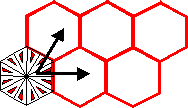The group of displacement is generated by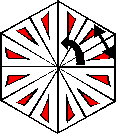The contour of the tile is a right triangle, the angles are 30°, 60° and 90°.